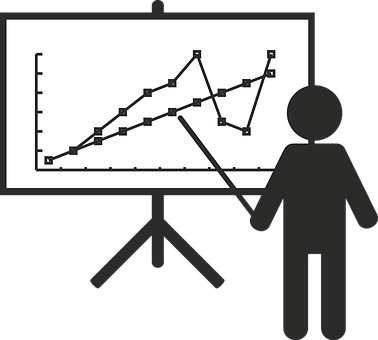📚

All Subjects

>

📊

AP Stats

>

✌️

Unit 2

# 2.1 Introducing Statistics: Are Variables Related?Peter Cao

### AP Statistics📊

Bookmarked 4.3k • 246 resources
See Unitsimage courtesy of: pixabay.com

## Types of Data

We start off things with a review of categorical and quantitative data. Categorical data is data that is an attribute that is usually represented with a percentage or proportion, while quantitative data is data that can be represented by a number. If data can be averaged, it's quantitative.

### Bivariate Categorical Data

When we collect data, we will record data from two different variables at the same time. Sometimes we have two categorical variables such as “class” and “does homework on time,” for example. We will want to know whether certain classes, such as juniors, finish their homework on time more than compared to other classes.
Bivariate categorical data can be represented using a histogram, frequency chart, or a mosaic plot.

### Bivariate Quantitative Data

Other times, we’ll have two sets of qualitative data such as plant height and amount of fertilizer used and we’ll want to see whether more fertilizer is correlated with a taller plant. This type of data is usually represented using a scatterplot.

## How Can I Use This Data?

In both cases, we are trying to see whether both variables are related to each other. If we know that two variables are related to each other, then we may be able to predict the behavior of another variable if we know the value of one variable. Keep in mind that finding that two variables do not influence each other can also be just as strong of a analytical discovery than finding that they do influence each other.
🎥Watch: AP Stats - Scatterplots and Association

## Resources:

Thousands of students are studying with us for the AP Statistics exam.
#####Studying with Hours = the ultimate focus mode
Start a free study session
##### 🔍 Are you ready for college apps?
Take this quiz and find out!
Start Quiz
Browse Study Guides By Unit
📆Big Reviews: Finals & Exam Prep
✏️Blogs
✍️Free Response Questions (FRQs)
👆Unit 1: Exploring One-Variable Data
✌️Unit 2: Exploring Two-Variable Data
🔎Unit 3: Collecting Data
🎲Unit 4: Probability, Random Variables, and Probability Distributions
📊Unit 5: Sampling Distributions
⚖️Unit 6: Inference for Categorical Data: Proportions
😼Unit 7: Inference for Qualitative Data: Means
✳️Unit 8: Inference for Categorical Data: Chi-Square
📈Unit 9: Inference for Quantitative Data: Slopes
#####FREE AP stats Survival Pack + Cram Chart PDF
Thousands of students are studying with us for the AP Statistics exam.